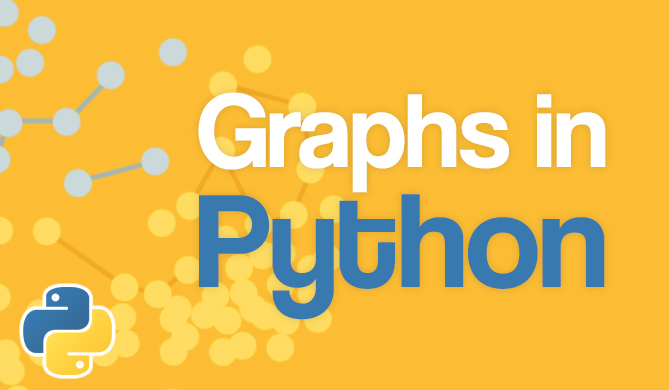Binary Search in JavaScript

# Binary Search in JavaScript## Introduction

Searching is one of the most commonly performed tasks in the domain of Computer Science. Many algorithms and data structures exist to make searching more efficient.

In this article, we'll look at the idea behind Binary Search and how to implement it in JavaScript.

Binary Search is a very simple, intuitive, yet efficient searching algorithm. The only caveat is that it works only sorted arrays, so some preprocessing on our data in order to sort it might be necessary.

## Understanding Binary Search

Binary Search is a divide-and-conquer algorithm, that divides the array roughly in half every time it checks whether an element of the array is the one we're looking for.

In other words, we divide the problem into simpler problems until it becomes simple enough to solve them directly. Let's assume we have a sorted array (in ascending order) and take a look at the steps of binary search:

1. Find the middle element of the given array.
2. Compare the middle element with the value we are looking for (called key).
• If the key is less than the middle element, search in the left half.
• If the key is more than the middle element, search in the right half.
• If the key is equal to the middle element, return the index of the middle element.
3. Continue with steps 1, 2 until we are left with a single element.

To understand this better, let's look at an example of why we can simply discard half of the current search range each time we check an element:

Similarly to this first split, we can keep dividing the array until we either find the element, or end up with only one candidate for the key.

In this case, we ended up with only one possible candidate for the key, and it turned out to match the element we were looking for.

As you can see in the example, it took us relatively few comparisons to find the element we needed. Namely, we only needed to do four comparisons to find the element in an array of 11 elements. We'll take a closer look at the efficiency of Binary Search later, but it should already be clear that it outclasses simple searching algorithms like Linear Search.

## Implementation of Binary Search in JavaScript

Now that we've gone through the logic behind Binary Search let us implement it in JavaScript:

Free eBook: Git Essentials

Check out our hands-on, practical guide to learning Git, with best-practices, industry-accepted standards, and included cheat sheet. Stop Googling Git commands and actually learn it!

function binarySearch(sortedArray, key){
let start = 0;
let end = sortedArray.length - 1;

while (start <= end) {
let middle = Math.floor((start + end) / 2);

if (sortedArray[middle] === key) {
// found the key
return middle;
} else if (sortedArray[middle] < key) {
// continue searching to the right
start = middle + 1;
} else {
// search searching to the left
end = middle - 1;
}
}
// key wasn't found
return -1;
}


Here, we are using two variables to keep track of the start and end of the current subarray we are searching. We find the middle element, and then check whether it is equal, lesser than, or greater than the key.

As explained previously, given that we have a sorted array, we can discard half of the elements in the array. We accomplish this in code by changing the start or end variable, depending on where we're continuing our search. If the current element we're looking at is less than the key, we change start to middle + 1 and effectively discard the current element and all elements less than that.

## The Efficiency of Binary Search

The time complexity of the Binary Search is O(log2n), where n is the number of elements in the array. This is far better compared to the Linear Search, which is of time complexity O(n). Like many other search algorithms, Binary Search is an in-place algorithm. That means that it works directly on the original array without making any copies.

We have to keep in mind however, that Binary Search only works on sorted arrays. The sorting step itself, if using an efficient sorting algorithm, has a complexity of O(nlogn). This means that in most cases, if the array is small, or if we need to search it only once, a brute-force (e.g. Linear Search) algorithm might be better.

Given this, Binary Search really shines when we need to make repeated searches on large arrays. As previously mentioned, we needed only 4 comparisons (comparisons being the most intensive tasks of all search algorithms), for an array of 11 elements. However, if we had an array of 10,000,000 elements, we would only need to check 24 elements, i.e. 0.0002% of the entire array.

## Conclusion

In this article, we have taken a look at Binary Search. It's simple, intuitive and efficient logic and implementation make it a very popular algorithm to demonstrate the divide-and-conquer strategy.

Last Updated: September 19th, 2023

### You might also like...

Get tutorials, guides, and dev jobs in your inbox.

Abhilash KakumanuAuthor

Hey, I am a full-stack web developer located in India. I am a curious person who is always trying to wrap my head around new technologies. In my free time, I read novels and play with my dog!Free
Course

### Graphs in Python - Theory and Implementation

# python# data structures# algorithms# computer science

Graphs are an extremely versatile data structure. More so than most people realize! Graphs can be used to model practically anything, given their nature of...DetailsProject

### React State Management with Redux and Redux-Toolkit

# javascript# React

Coordinating state and keeping components in sync can be tricky. If components rely on the same data but do not communicate with each other when...Details